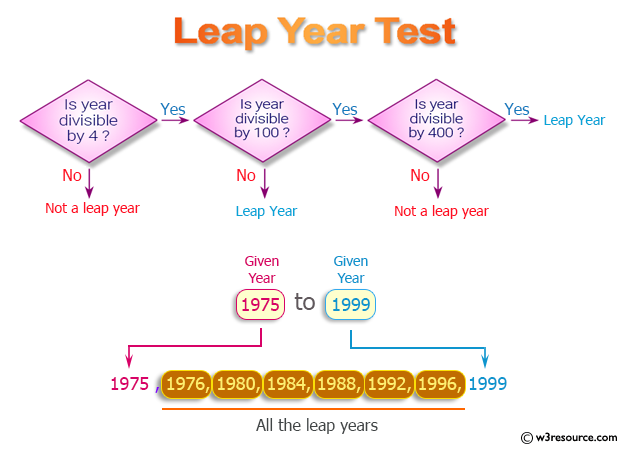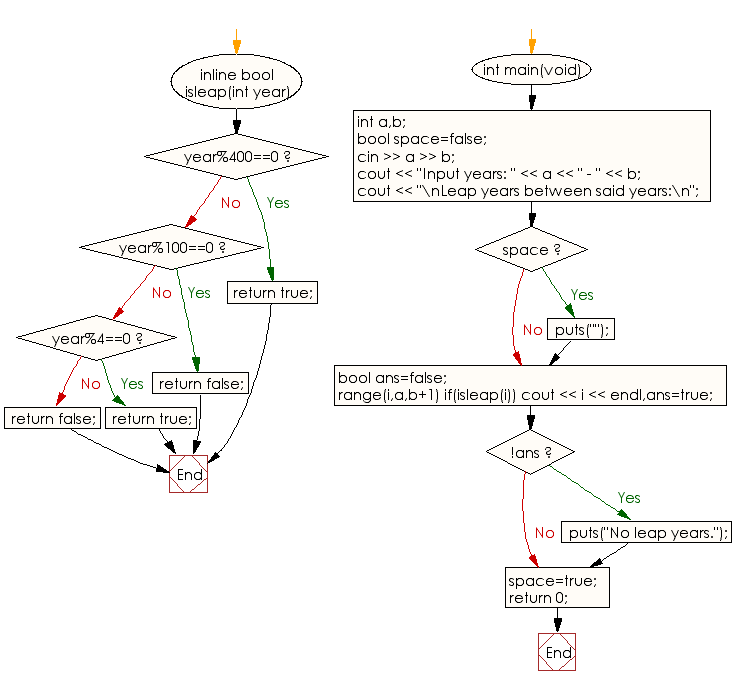﻿ C++ : Display all the leap years between two given years

# C++ Exercises: Display all the leap years between two given years

## C++ Basic: Exercise-79 with Solution

Write a C++ program to display all the leap years between two given years. If there is no leap year in the given period,display a suitable message.
Note: Range of the two given years: ( 0 < year1 ≤ year2 < 3,000).

Pictorial Presentation:Sample Solution:

C++ Code :

``````#include <iostream>
#define range(i,a,b) for(int (i)=(a);(i)<(b);(i)++)
#define rep(i,n) range(i,0,n)
using namespace std;

inline bool isleap(int year){
if(year%400==0)
return true;
if(year%100==0)
return false;
if(year%4==0)
return true;
return false;
}

int main(void){
int a,b;
bool space=false;
cin >> a >> b;
cout << "Input years: " << a << " - " << b;
cout << "\nLeap years between said years:\n";
if(space) puts("");
bool ans=false;
range(i,a,b+1) if(isleap(i)) cout << i << endl,ans=true;
if(!ans) puts("No leap years.");
space=true;

return 0;
}
``````

Sample Output:

```Input years: 1975 - 2018
Leap years between said years:
1976
1980
1984
1988
1992
1996
2000
2004
2008
2012
2016
```

Flowchart:C++ Code Editor:

What is the difficulty level of this exercise?

﻿

## C++ Programming: Tips of the Day

Why is there no std::stou?

The most pat answer would be that the C library has no corresponding "strtou", and the C++11 string functions are all just thinly veiled wrappers around the C library functions: The std::sto* functions mirror strto*, and the std::to_string functions use sprintf.

Ref: https://bit.ly/3wtz2qA

We are closing our Disqus commenting system for some maintenanace issues. You may write to us at reach[at]yahoo[dot]com or visit us at Facebook